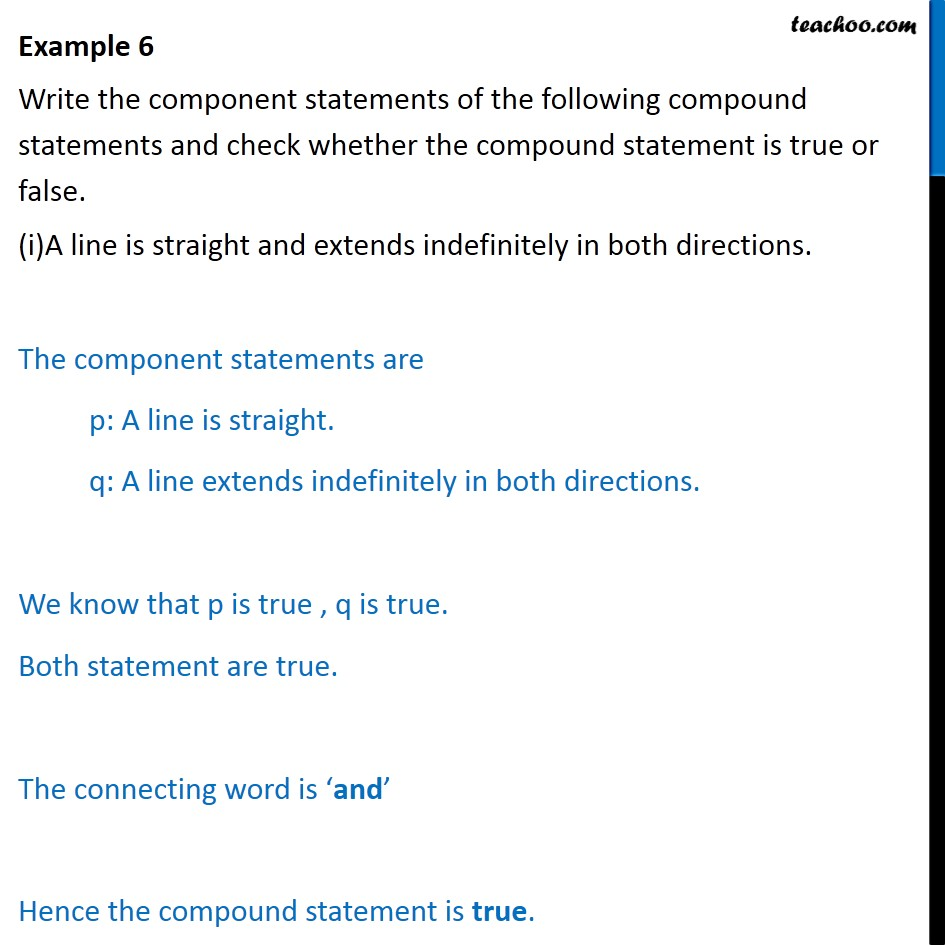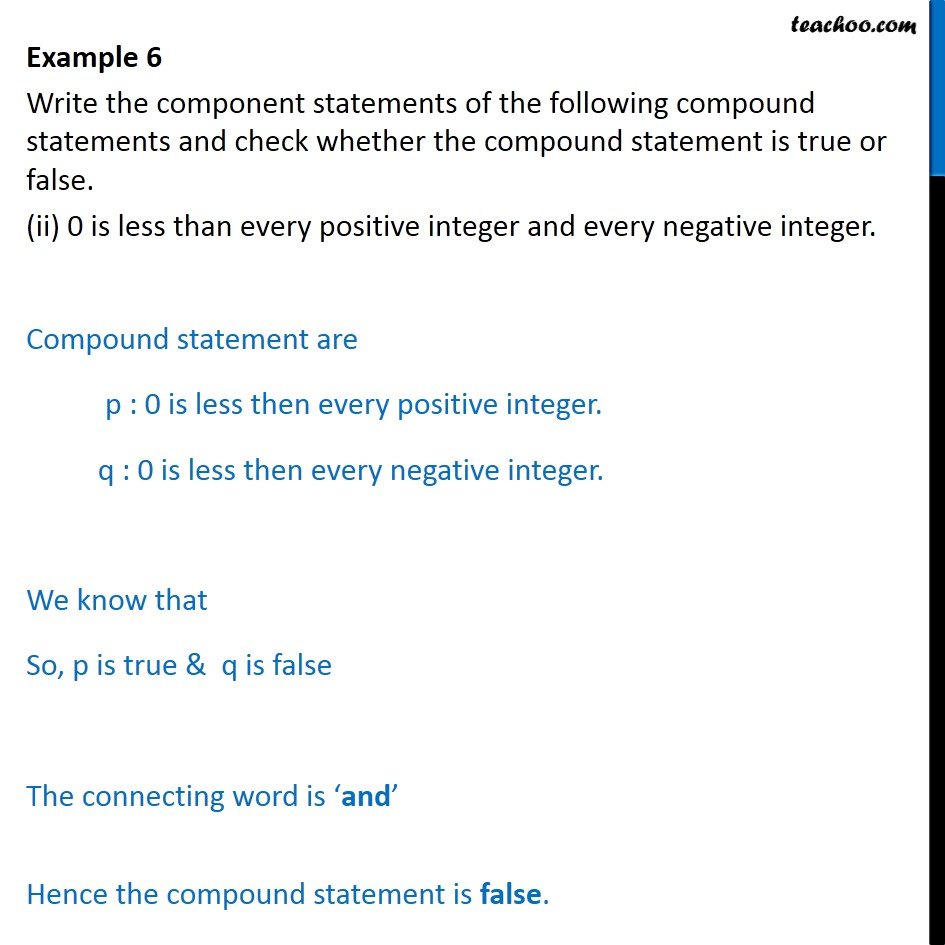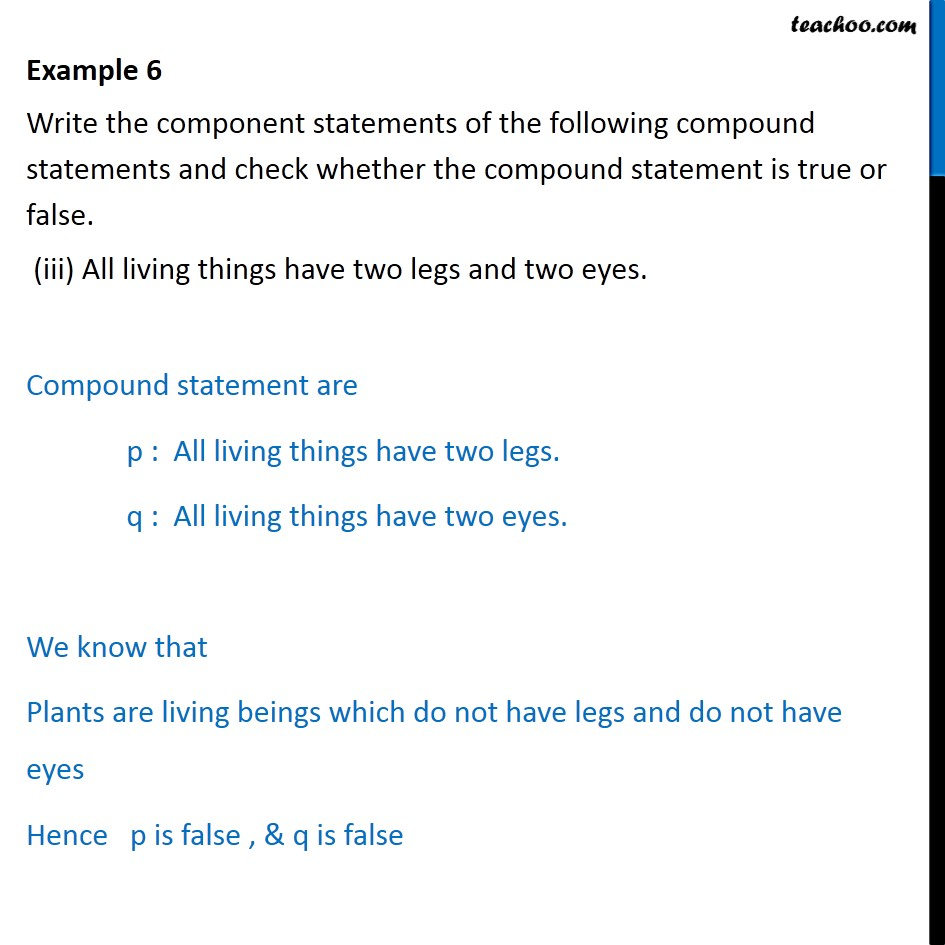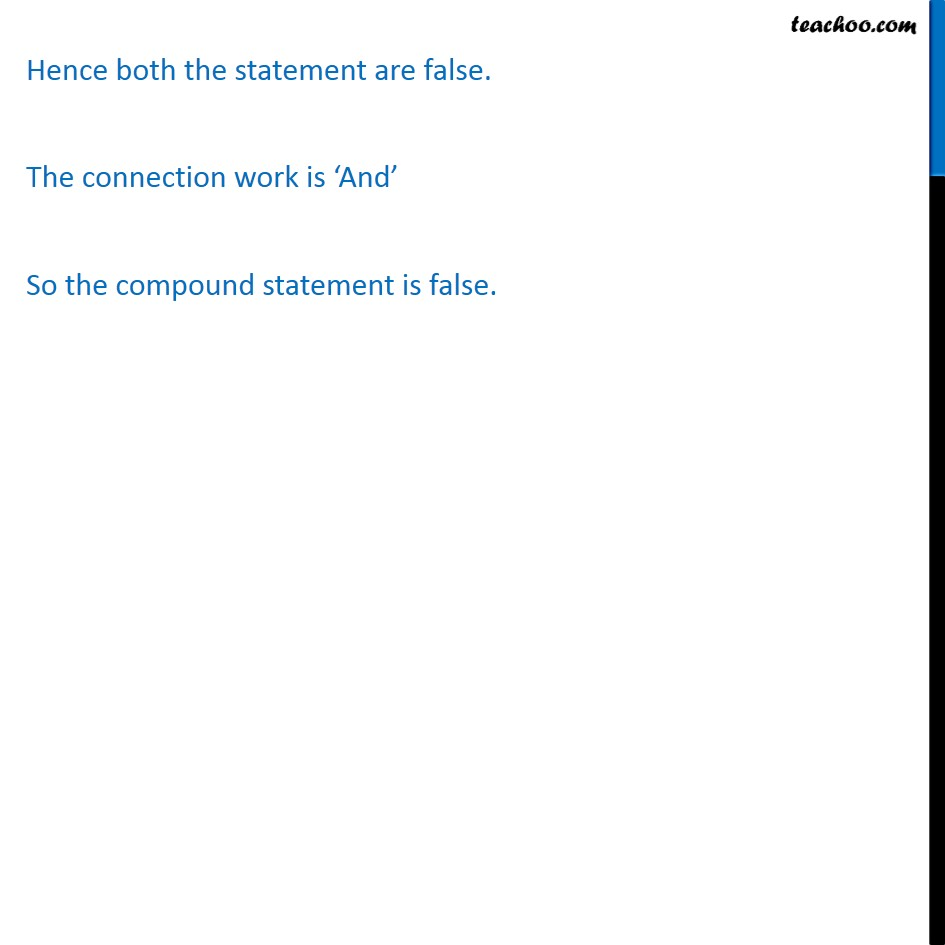Subscribe to our Youtube Channel - https://you.tube/teachoo

1. Chapter 14 Class 11 Mathematical Reasoning
2. Concept wise
3. Words 'And' & 'Or'

Transcript

Example 6 Write the component statements of the following compound statements and check whether the compound statement is true or false. (i)A line is straight and extends indefinitely in both directions. The component statements are p: A line is straight. q: A line extends indefinitely in both directions. We know that p is true , q is true. Both statement are true. The connecting word is and Hence the compound statement is true. Example 6 Write the component statements of the following compound statements and check whether the compound statement is true or false. (ii) 0 is less than every positive integer and every negative integer. Compound statement are p : 0 is less then every positive integer. q : 0 is less then every negative integer. We know that So, p is true & q is false The connecting word is and Hence the compound statement is false. Example 6 Write the component statements of the following compound statements and check whether the compound statement is true or false. (iii) All living things have two legs and two eyes. Compound statement are p : All living things have two legs. q : All living things have two eyes. We know that Plants are living beings which do not have legs and do not have eyes Hence p is false , & q is false Hence both the statement are false. The connection work is And So the compound statement is false.

Words 'And' & 'Or'